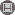Volume 14, Issue 2 (10-2019)                   IJMSI 2019, 14(2): 105-125 | Back to browse issues page

BibTeX | RIS | EndNote | Medlars | ProCite | Reference Manager | RefWorks
Send citation to:Ramane H, Gudodagi G, Manjalapur V, Alhevaz A. On Complementary Distance Signless Laplacian Spectral Radius and Energy of Graphs. IJMSI. 2019; 14 (2) :105-125
URL: http://ijmsi.ir/article-1-1017-en.html
Abstract:

Let \$D\$ be a diameter and \$d_G(v_i, v_j)\$ be the distance between the vertices \$v_i\$ and \$v_j\$ of a connected graph \$G\$. The complementary distance signless Laplacian matrix of a graph \$G\$ is \$CDL^+(G)=[c_{ij}]\$ in which \$c_{ij}=1+D-d_G(v_i, v_j)\$ if \$ineq j\$ and \$c_{ii}=sum_{j=1}^{n}(1+D-d_G(v_i, v_j))\$. The complementary transmission \$CT_G(v)\$ of a vertex \$v\$ is defined as \$CT_G(v)=sum_{u in V(G)}[1+D-d_G(u, v)]\$. Let \$CT(G)=diag[CT_G(v_1), CT_G(v_2), ldots, CT_G(v_n)]\$. The complementary distance signless Laplacian matrix of \$G\$ is \$CDL^+(G)=CT(G)+CD(G)\$.

If \$rho_1, rho_2, ldots, rho_n\$ are the eigenvalues of \$CDL^+(G)\$ then the complementary distance signless Laplacian energy of \$G\$ is defined as \$E_{CDL^+}(G)=sum_{i=1}^{n}left| rho_i-frac{1}{n}sum_{j=1}^{n}CT_G(v_j)right|\$.
noindent
In this paper we obtain the bounds for the largest eigenvalue of \$CDL^+(G)\$. Further we determine Nordhaus-Gaddum type results for the largest eigenvalue. In the sequel we establish the bounds for the complementary distance signless Laplacian energy.}

Type of Study: Research paper | Subject: Special